# Solution assignment 10 Quadratic functions and graphs

### Assignment 10

For which value of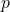do the parabolas: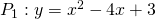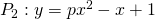have just one point in common.

### Solution

In the common pointsandthe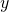-coordinates are equal. In order to find the corresponding-coordinates also the right-hand sides ofenhave to be equal: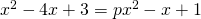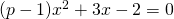This equation has only one solution (actually two coinciding solutions) if: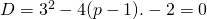Thus the two parabolas have only one common point if: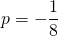0